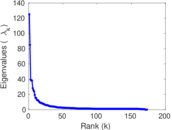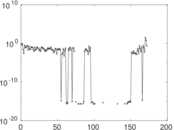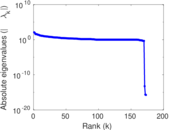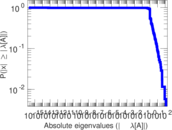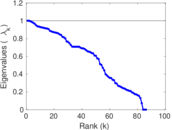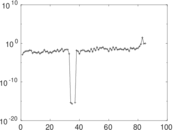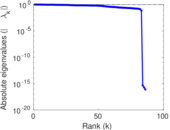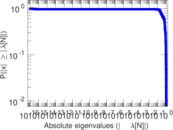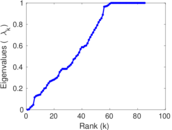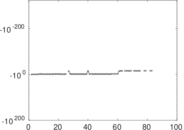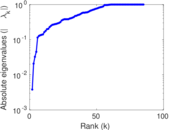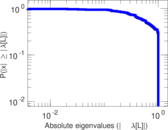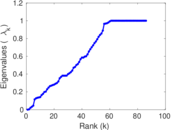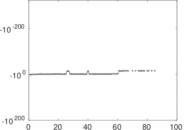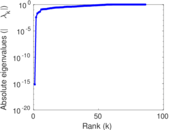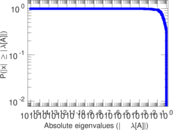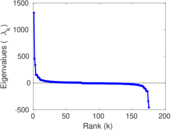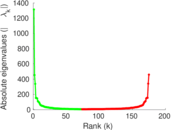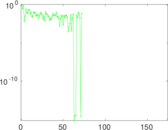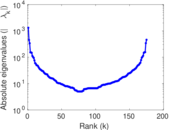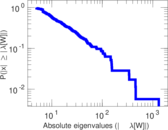# Wiktionary edits (rw)

This is the bipartite edit network of the Kinyarwanda Wiktionary. It contains users and pages from the Kinyarwanda Wiktionary, connected by edit events. Each edge represents an edit. The dataset includes the timestamp of each edit.

 Code `mrw` Internal name `edit-rwwiktionary` Name Wiktionary edits (rw) Data source http://dumps.wikimedia.org/ AvailabilityDataset is available for download Consistency checkDataset passed all tests Category Authorship network Dataset timestamp 2017-10-20 Node meaning User, article Edge meaning Edit Network formatBipartite, undirected Edge typeUnweighted, multiple edges Temporal dataEdges are annotated with timestamps

## Statistics

 Size n = 1,321 Left size n1 = 175 Right size n2 = 1,146 Volume m = 6,776 Unique edge count m̿ = 3,268 Wedge count s = 274,827 Claw count z = 19,620,161 Cross count x = 1,233,074,874 Square count q = 456,519 4-Tour count T4 = 4,758,304 Maximum degree dmax = 1,019 Maximum left degree d1max = 1,019 Maximum right degree d2max = 66 Average degree d = 10.258 9 Average left degree d1 = 38.720 0 Average right degree d2 = 5.912 74 Fill p = 0.016 295 2 Average edge multiplicity m̃ = 2.073 44 Size of LCC N = 990 Diameter δ = 18 50-Percentile effective diameter δ0.5 = 4.060 52 90-Percentile effective diameter δ0.9 = 8.054 96 Median distance δM = 5 Mean distance δm = 5.152 73 Gini coefficient G = 0.775 089 Balanced inequality ratio P = 0.189 787 Left balanced inequality ratio P1 = 0.102 568 Right balanced inequality ratio P2 = 0.234 504 Relative edge distribution entropy Her = 0.794 703 Power law exponent γ = 2.453 36 Tail power law exponent γt = 1.881 00 Tail power law exponent with p γ3 = 1.881 00 p-value p = 0.000 00 Left tail power law exponent with p γ3,1 = 1.661 00 Left p-value p1 = 0.010 000 0 Right tail power law exponent with p γ3,2 = 1.931 00 Right p-value p2 = 0.000 00 Degree assortativity ρ = +0.241 431 Degree assortativity p-value pρ = 1.471 05 × 10−44 Spectral norm α = 125.151 Algebraic connectivity a = 0.003 797 35 Spectral separation |λ1[A] / λ2[A]| = 1.472 41 Controllability C = 961 Relative controllability Cr = 0.738 663

## Plots

### Fruchterman–Reingold graph drawing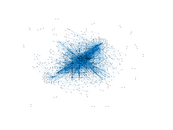### Degree distribution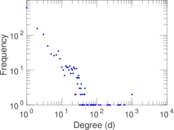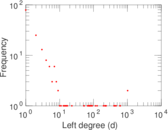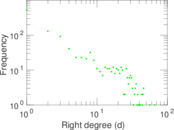### Cumulative degree distribution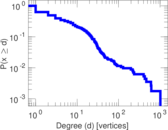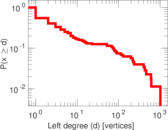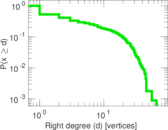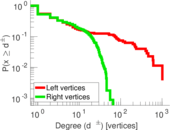### Lorenz curve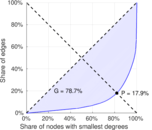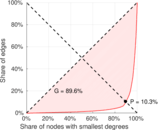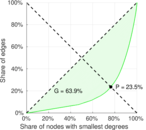### Spectral distribution of the adjacency matrix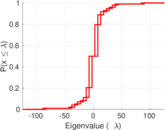### Spectral distribution of the normalized adjacency matrix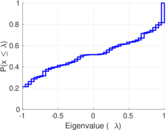### Spectral distribution of the Laplacian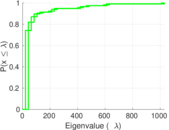### Spectral graph drawing based on the adjacency matrix### Spectral graph drawing based on the Laplacian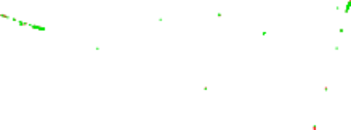### Spectral graph drawing based on the normalized adjacency matrix### Degree assortativity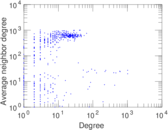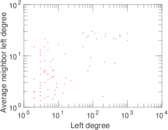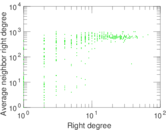### Zipf plot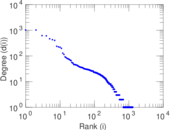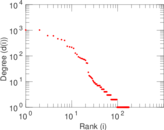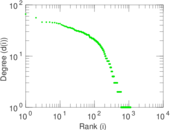### Hop distribution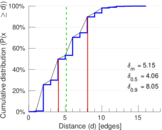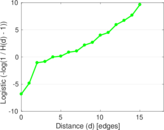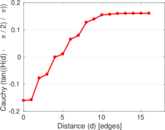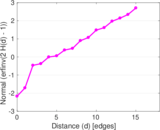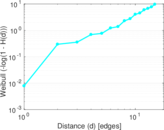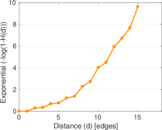### Double Laplacian graph drawing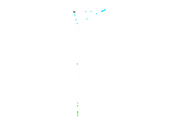### Delaunay graph drawing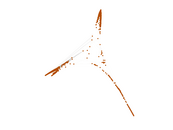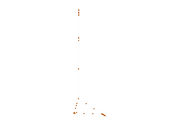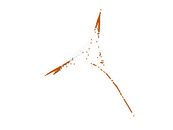### Edge weight/multiplicity distribution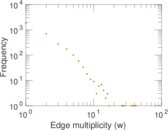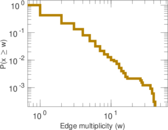### Temporal distribution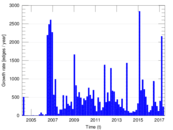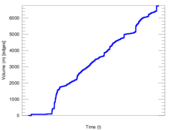### Temporal hop distribution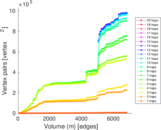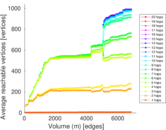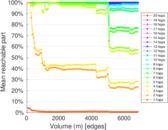### Diameter/density evolution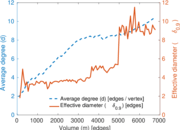### Matrix decompositions plots